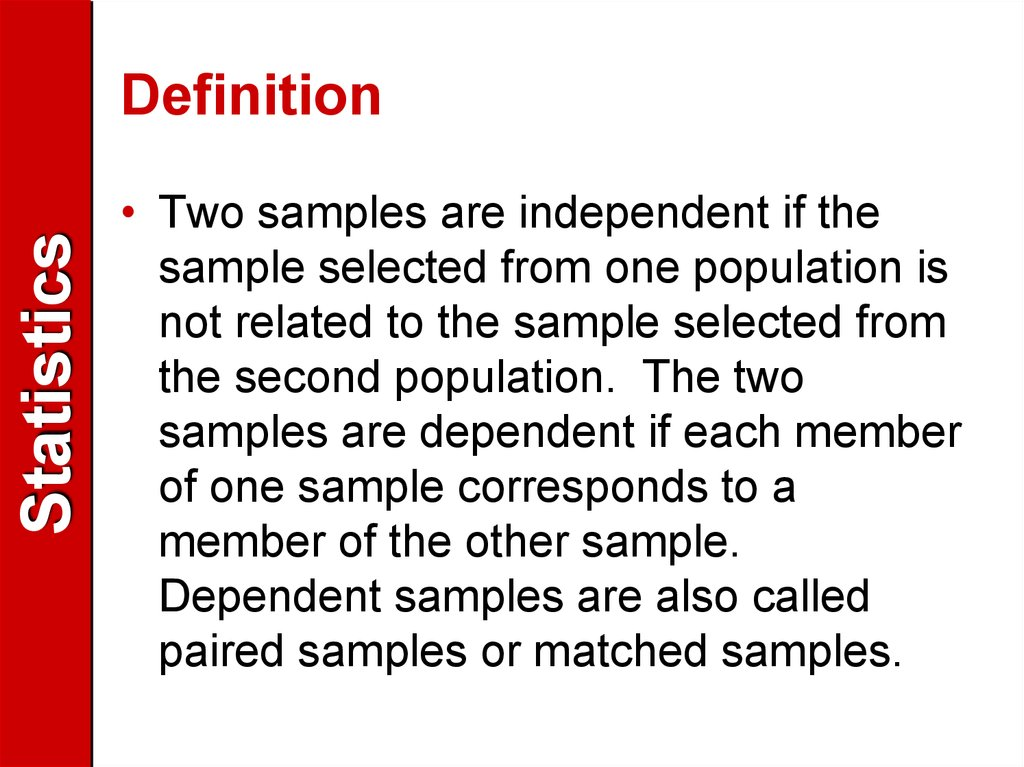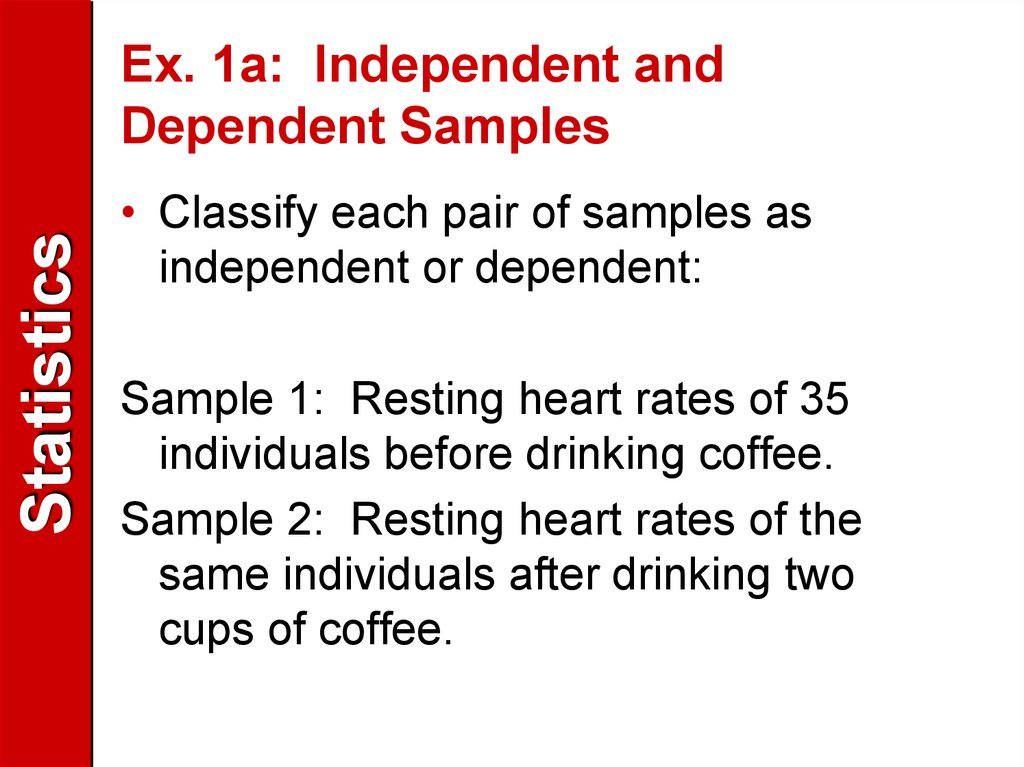# Definition. Statistics

## 1. Definition

Statistics
Definition
• Two samples are independent if the
sample selected from one population is
not related to the sample selected from
the second population. The two
samples are dependent if each member
of one sample corresponds to a
member of the other sample.
Dependent samples are also called
paired samples or matched samples.

## 2. Ex. 1a: Independent and Dependent Samples

Statistics
Ex. 1a: Independent and
Dependent Samples
• Classify each pair of samples as
independent or dependent:
Sample 1: Resting heart rates of 35
individuals before drinking coffee.
Sample 2: Resting heart rates of the
same individuals after drinking two
cups of coffee.

## 3. Ex. 1: Independent and Dependent Samples

Statistics
Ex. 1: Independent and
Dependent Samples
Sample 1: Resting heart rates of 35 individuals
before drinking coffee.
Sample 2: Resting heart rates of the same
individuals after drinking two cups of coffee.
These samples are dependent. Because the
resting heart rates of the same individuals
were taken, the samples are related. The
samples can be paired with respect to each
individual.

## 4. Ex. 1b: Independent and Dependent Samples

Statistics
Ex. 1b: Independent and
Dependent Samples
• Classify each pair of samples as
independent or dependent:
Sample 1: Test scores for 35 statistics
students
Sample 2: Test scores for 42 biology
students who do not study statistics

## 5. Ex. 1b: Independent and Dependent Samples

Statistics
Ex. 1b: Independent and
Dependent Samples
Sample 1: Test scores for 35 statistics
students
Sample 2: Test scores for 42 biology students
who do not study statistics
These samples are independent. It is not
possible to form a pairing between the
members of samples—the sample sizes are
different and the data represent test scores
for different individuals.

## 6. Note:

Statistics
Note:
Dependent samples often involve
identical twins, before and after results
for the same person or object, or results
of individuals matched for specific
characteristics.

## 7. The t-Test for the Difference Between Means

Statistics
The t-Test for the Difference
Between Means
• To perform a two-sample hypothesis
test with xdependent
samples, you will
1 x2
use a different technique. You will first
find the difference for each data pair,
. The test statistic is the mean
of these differences,
d x1 x2
d ( d ) / n

## 8. To conduct the test, the following conditions are required:

Statistics
To conduct the test, the following
conditions are required:
• The samples must be dependent
(paired) and randomly selected.
• Both populations must be normally
distributed.
If these two requirements are met, then
the sampling distribution for d , the
mean of the differences of the paired
data entries in the dependent samples,

## 9. To conduct the test, the following conditions are required:

Statistics
To conduct the test, the following
conditions are required:
• has a t-distribution with n – 1 degrees
of freedom, where n is the number of
data pairs.

## 10.

Statistics
The following symbols are used for the t-test for d.
Although formulas are given for the mean and standard deviation of
differences, we suggest you use a technology tool to calculate
these statistics.

## 11. Because the sampling distribution for is a t-distribution, you can use a t-test to test a claim about the mean of the differences for a population of paired data.

Statistics
Because the sampling distribution for d is a t-distribution,
you can use a t-test to test a claim about the mean of the
differences for a population of paired data.
STUDY TIP: If n > 29, use the last row (∞) in
the t-distribution table.

Statistics

## 13. Ex. 2: The t-Test for the Difference Between Means

Statistics
Ex. 2: The t-Test for the Difference
Between Means
• A golf club manufacturer claims that golfers
can lower their score by using the
manufacturer’s newly designed golf clubs.
Eight golfers are randomly selected and each
is asked to give his or her most recent score.
After using the new clubs for one month, the
golfers are again asked to give their most
recent scores. The scores for each golfer
are given in the next slide. Assuming the golf
scores are normally distributed, is there
enough evidence to support the
manufacturer’s claim at = 0.10?

## 14.

Statistics
• The claim is that “golfers can lower their
scores.” In other words, the manufacturer
claims that the score using the old clubs will be
greater than the score using the new clubs.
Each difference is given by:
d = (old score) – (new score)
The null and alternative hypotheses are
Ho: d 0
and Ha: d > 0 (claim)

## 15. Because the test is a right-tailed test,  = 0.10, and d.f. = 8 – 1 = 7, the critical value for t is 1.415. The rejection region is t > 1.415. Using the table below, you can calculate and sd as follows:

Statistics
Because the test is a right-tailed test, = 0.10, and d.f. = 8 – 1
= 7, the critical value for t is 1.415. The rejection region is t >
1.415. Using the table below, you can calculate d and sd as
follows:
d 13
d
1.625
n
8
n( d ) ( d )
2
sd
2
n(n 1)
8(87) (13)
sd
3.07
8(8 1)
2

## 16. Using the t-test, the standardized test statistic is:

Statistics
Using the t-test, the standardized
test statistic is:
d ud
t
sd / n
1.625 0
t
1.50
3.07
8
• The graph below shows the
location of the rejection
region and the standardized
test statistic, t. Because t is
in the rejection region, you
should decide to reject the
null hypothesis. There is not
enough evidence to support
the golf manufacturer’s claim
at the 10% level The results
of this test indicate that after
using the new clubs, golf
scores were significantly
lower.

## 17. Ex. 3: The t-Test for the Difference Between Means

Statistics
Ex. 3: The t-Test for the Difference
Between Means
• A state legislator wants to determine whether
her voter’s performance rating (0-100) has
changed from last year to this year. The
following table shows the legislator’s
performance rating for the same 16 randomly
selected voters for last year and this year. At
= 0.01, is there enough evidence to
conclude that the legislator’s performance
rating has changed? Assume the
performance ratings are normally distributed.

## 18.

Statistics
• If there is a change in the legislator’s rating,
there will be a difference between “this year’s”
ratings and “last year’s) ratings. Because the
legislator wants to see if there is a difference,
the null and alternative hypotheses are:
Ho: d = 0
and Ha: d 0 (claim)

## 19. Because the test is a tw0-tailed test,  = 0.01, and d.f. = 16 – 1 = 15, the critical values for t are 2.947. The rejection region are t < -2.947 and t > 2.947.

Statistics
Because the test is a tw0-tailed test, = 0.01, and d.f. = 16 – 1
= 15, the critical values for t are 2.947. The rejection region
are t < -2.947 and t > 2.947.
d 53
d
3.3125
n
16
n( d ) ( d )
2
sd
2
n(n 1)
16(1581) (53)
sd
9.68
16(16 1)
2

## 20. Using the t-test, the standardized test statistic is:

Statistics
Using the t-test, the standardized
test statistic is:
d ud t 3.3125 0 1.369
t
9.68
sd / n
• The graph shows the
location of the rejection
region and the standardized
test statistic, t. Because t is
not in the rejection region,
you should fail to reject the
null hypothesis at the 1%
level. There is not enough
evidence to conclude that
the legislator’s approval
rating has changed.
16

## 21. Using Technology

Statistics
Using Technology
• If you prefer to use a
technology tool for this
type of test, enter the
data in two columns
and form a third column
in which you calculate
the difference for each
pair. You can now
perform a one-sample
t-test on the difference
column as shown in
Chapter 7.
• Stat|Edit|enter data
• Subtract L1 – L2 = in
L3.
• STAT|Tests|t-test
• Data
• =0
• List: L3
• Freq: 1
• 0
• Calculate

## 22. Using Technology

Statistics
Using Technology
Stat|Edit|enter data
Subtract L1 – L2 = in L3.
STAT|Tests|t-test
Data
=0
List: L3
Freq: 1
0
Calculate
• 0
• T = 1.369 (standardized test
statistic)
• P = don’t worry about it
• X bar = 3.3125 – same as d
bar.
• Sx = 9.68 which is Sd
I find it easy to draw and enter
the data into the curve part
so I can visually see the
rejection region. You will